# Test: Square Root And Cube Root- 1

## 10 Questions MCQ Test Quantitative Aptitude for GMAT | Test: Square Root And Cube Root- 1

Description
Attempt Test: Square Root And Cube Root- 1 | 10 questions in 20 minutes | Mock test for Quant preparation | Free important questions MCQ to study Quantitative Aptitude for GMAT for Quant Exam | Download free PDF with solutions
QUESTION: 1

###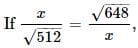find the value of x.

Solution: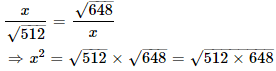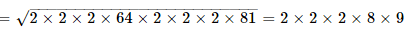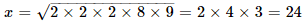QUESTION: 2

###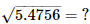Solution: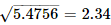QUESTION: 3

###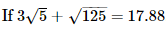then what will be the value of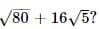Solution: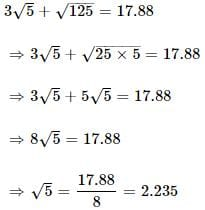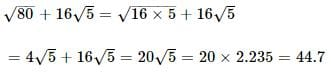QUESTION: 4

The cube root of 0.000729 is

Solution: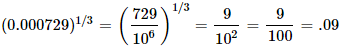QUESTION: 5

What is the least perfect square which is divisible by each of 21, 36 and 66?

Solution:

LCM of 21, 36, 66 = 2772
i.e., all multiples of 2772 are divisible by 21, 36 and 66
Prime factorization of 2772 is,
2772 = 2 × 2 × 3 × 3 × 7 × 11
i.e., to make it a perfect square, we have to multiply it by 7 and 11
Hence, required number = 2772 × 7 × 11 = 213444

QUESTION: 6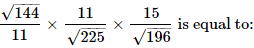Solution: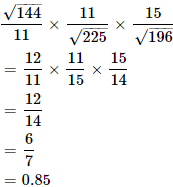QUESTION: 7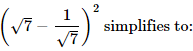Solution:

The correct option is Option C.

(√7 - 1/√7)²

= (√7)² - 2 × √7 × 1/√7 + (1/√7)²

= 7 - 2 + 1/7

= 5 + 1/7

= 36/7

QUESTION: 8

The square root of 16641 is

Solution: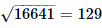QUESTION: 9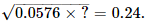Solution: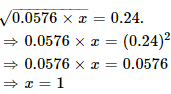QUESTION: 10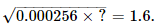Solution: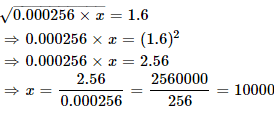Use Code STAYHOME200 and get INR 200 additional OFF Use Coupon Code